NCERT Solutions: Probability (Exercise 14.1) - 1

# Probability (Exercise 14.1) - 1 NCERT Solutions - Mathematics (Maths) Class 10

Q1. Complete the following statements.
(i) Probability of an event E + Probability of the event ‘not E’ =  ________
(ii) The probability of an event that cannot happen is  ________. Such an event is called  ________.
(iii) The probability of an event that is certain to happen is  ________ Such an event is called  ________.
(iv) The sum of the probabilities of all the elementary events of an experiment is  ________.
(v) The probability of an event is greater than or equal to  ________ and less than or equal to  ________.

Ans:
(i) Probability of an event E + Probability of the event 'not E' = 1.
(ii) The probability of an event that cannot happen is 0. Such an event is called an impossible event.
(iii) The probability of an event that is certain to happen is 1. Such an event is called a sure or certain event.
(iv) The sum of the probabilities of all the elementary events of an experiment is 1.
(v) The probability of an event is greater than or equal to 0 and less than or equal to 1.

Q2. Which of the following experiments have equally likely outcomes? Explain.
(i) A driver attempts to start a car. The car starts or does not start.
(ii) A player attempts to shoot a basketball. She/he shoots or misses the shot.
(iv) A baby is born. It is a boy or a girl.

Ans:
(i) Since the car may or may not start, thus the outcomes are not equally likely.
(ii) The player may shoot or miss the shot.
∴ The outcomes are not equally likely.
(iii) In advance, it is known that the answer is to be either right or wrong.
The outcomes right or wrong are equally likely to occur.
(iv) In advance, it is known the newly born baby has to be either a boy or a girl.
∴ The outcomes, either a boy or a girl are equally likely to occur.

Q3. Why is tossing a coin considered to be a fair way of deciding which team should get the ball at the beginning of a football game?
Ans: Since on tossing a coin, the outcomes ‘head’ and ‘tail’ are equally likely, the result of tossing a coin is completely unpredictable and so it is a fairway.

Q4. Which of the following cannot be the probability of an event?
(a) 2/3
(b) − 1.5
(c) 15%
(d) 0.7

Ans: (b)
Solution: Since the probability of an event cannot be negative.
Option (b) −1.5 cannot be the probability of an event.

Q5. If P(E) = 0.05, what is the probability of ‘not E’?
Ans: We know that,

P(E) + P(not E) = 1
∴ 0.05 + P(not E) = 1 ⇒ P(not E) = 1 − 0.05
= 0.95
Thus, the probability of ‘not E’ = 0.95.

Q6. A bag contains lemon flavoured candies only. Malini takes out one candy without looking into the bag. What is the probability that she takes out
(i) an orange flavoured candy?
(ii) a lemon flavoured candy?

Ans:
(i) Since, there are lemon flavoured candies only in the bag,
∴ Taking out any orange flavoured candy is not possible.
⇒ Probability of taking out an orange flavoured candy = 0.
(ii) Also, the probability of taking out a lemon flavoured candy = 1.

Q7. It is given that in a group of 3 students, the probability of 2 students not having the same birthday is 0.992. What is the probability that the 2 students have the same birthday?
Ans: ∴ Let the probability of 2 students having same birthday = P(SB) And the probability of 2 students not having the same birthday = P(nSB)
∴ P(SB) + P(nSB)=1
⇒ P(SB) + 0.992 = 1
⇒ P(SB)=1 − 0.992 = 0.008
So, the required probability of 2 boys having the same birthday = 0.008.

Q8. A bag contains 3 red balls and 5 black balls. A ball is drawn at random from the bag. What is the probability that the ball drawn is (i) red? (ii) not red?
Ans:
Total number of balls = 3 + 5 = 8
∴ Number of all possible outcomes = 8
(i) For red balls
There are 3 red balls.
∴ Number of favourable outcomes = 3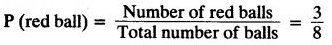(ii) For not red balls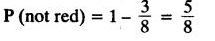Q9. A box contains 5 red marbles, 8 white marbles and 4 green marbles. One marble is taken out of the box at random. What is the probability that the marble taken out will be (i) red? and (ii) white? (iii) not green?
Ans:
Total number of marbles = 5 + 8 + 4 = 17
(i) For red marbles
∵ Number of red marbles = 5
∴ Number of favourable outcomes = 5
∴ Probability of red marbles, P(red) = 5/17
(ii) For white balls
∵ Number of white balls = 8
∴ Probability of white balls,
∴ P(white)= 8/17
(iii) For not green balls
∵ Number of white balls = 4
∴ Number of ‘not green’ balls = 17 − 4 = 13
i.e., Favourable outcomes = 13
P(not green) = 13/17
OR
Number of green marbles = 4
∴ Number of ‘not green balls’ = 17 − 4 = 13
⇒ Favourable outcomes = 13
∴ P(not green) = 13/17

Q10. A piggy bank contains hundred 50p coins, fifty Rs 1 coins, twenty Rs 2 coins and ten Rs 5 coins. If it is equally likely that one of the coins will fall out when the bank is turned upside down, what is the probability that the coin (i) will be a 50p coin? and (ii) will not be Rs 5 coin?
Ans:
Number of:
50 p coins = 100
Re 1 coins = 50
Rs 2 coins = 20
Rs 5 coins = 10
Total number of coins = 100 + 50 + 20 + 5 = 180
(i) For a 50 p coin:
Favourable events = 100
∴ P(50 p) = 100/180 = 5/9
(ii) For not a Rs 5 coin:
a Number of Rs 5 coins = 10
∴ Number of ‘not Rs 5’ coins = 180 − 10 = 170
⇒ Favourable outcomes = 170
∴ P(not 5 rupee coin) = 170/180 = 17/18.

Q11. Gopi buys a fish from a shop for his aquarium. The shopkeeper takes out one fish at random from a tank containing 5 male fish and 8 female fish (see Fig.). What is the probability that the fish taken out is a male fish?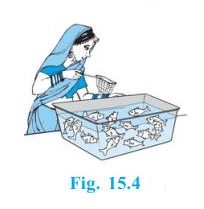Ans:
Number of:
Male fishes = 5
Female fishes = 8
∴ Total number of fishes = 5 + 8 = 13
⇒ Total number of outcomes = 13
For a male fish:
Number of favourable outcomes = 5
∴ P(male fish) = 5/13.

Q12. A game of chance consists of spinning an arrow which comes to rest pointing at one of the numbers 1, 2, 3, 4, 5, 6, 7, 8 (see figure), and these are equally likely outcomes. What is the probability that it will point at
(i) 8?
(ii) an odd number?
(iii) a number greater than 2?
(iv) a number less than 9?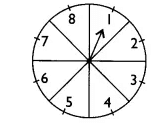Ans: Total numbers marked = 8
(i) When pointer points at 8
Total number of outcomes = 8
Number of favourable outcomes = 1
∴ P(8)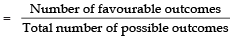= 1/8

(ii) When pointer points at an odd number
Number of odd numbers from 1 to 8 = 4
[∵ Odd numbers are 1, 3, 5 and 7]
⇒ Number of favourable outcomes = 4
∴ P(odd) =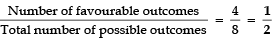(iii) When pointer points at a number greater than 2
Number of numbers greater than 2 = 6
[∴ The numbers 2, 3, 4, 5, 6, 7 and 8 are greater than 2]
⇒ Number of favourable outcomes = 6

∴ P(greater than 2) =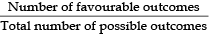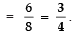(iv) When pointer points a number less than 9:
Number of numbers less than 9 = 8
[a The numbers 1, 2, 3, 4, 5, 6, 7 and 8 are less than 9]
∴ Number of favourable outcomes = 8

P(greater than 9)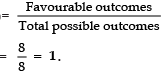Q13. A die is thrown once. Find the probability of getting
(i) a prime number.
(ii) a number lying between 2 and 6.
(iii) an odd number.

Ans: Since, numbers on a die are 1, 2, 3, 4, 5, and 6.
∴ Number of total outcomes = 6
(i) For prime numbers
Since 2, 3, and 5 are prime numbers,
∴ Favourable outcomes = 3
P(prime) =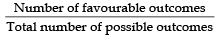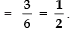(ii) For a number lying between 2 and 6
Since the numbers between 2 and 6 are 3, 4 and 5
∴ Favourable outcomes = 3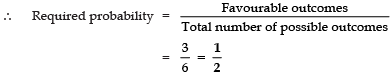(iii) For an odd number
Since 1, 3 and 5 are odd numbers.
⇒ Favourable outcomes = 3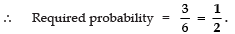Q14. One card is drawn from a well-shuffled deck of 52 cards. Find the probability of getting
(i) a king of red colour
(ii) a face card
(iii) a red face card
(iv) the jack of hearts
(vi) the queen of diamonds

Ans: Number of cards in deck = 52
∴ Total number of possible outcomes = 52
(i) For a king of red colour
∵ Number of red colour kings = 2
[∵ Kings of diamond and heart are red]
∴ Number of favourable outcomes = 2
E(red king)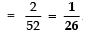(ii) For a face card
∵ 4 kings, 4 queens and 4 jacks are face cards
∴ Number of face cards = 12
⇒ Number of favourable outcomes = 12
∴ P(face)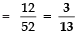(iii) For a red face card
Since cards of diamond and heart are red
∴ There are [2 kings, 2 queens, 2 jacks] 6 cards are red
⇒ Favourable outcomes = 6
∴ P(red face)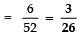(iv) For a jack of hearts
Since there is only 1 jack of hearts.
∴ Number of favourable outcomes = 1
P(jack of hearts)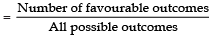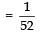∵There are 13 spades in a pack of 52 cards:
∴ Favourable outcomes are 13.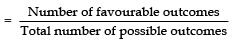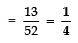(vi) For the queen of diamonds
∵ There is only one queen of a diamond.
∴ Number of favourable outcomes = 1
P(queen of diamonds)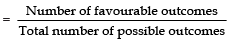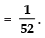The document Probability (Exercise 14.1) - 1 NCERT Solutions | Mathematics (Maths) Class 10 is a part of the Class 10 Course Mathematics (Maths) Class 10.
All you need of Class 10 at this link: Class 10

## Mathematics (Maths) Class 10

115 videos|479 docs|129 tests

## FAQs on Probability (Exercise 14.1) - 1 NCERT Solutions - Mathematics (Maths) Class 10

 1. What is probability and how is it related to the NCERT Solutions?Ans. Probability is a branch of mathematics that deals with the likelihood of an event occurring. In the context of the NCERT Solutions, probability is an important topic covered in Exercise 15.1. The solutions provide a comprehensive understanding of probability and its application in solving problems.
 2. How can I use the NCERT Solutions for Probability to prepare for my exams?Ans. The NCERT Solutions for Probability in Exercise 15.1 can be used as a valuable resource for exam preparation. By studying the solutions, you can gain a clear understanding of the concepts and techniques involved in probability. Additionally, practicing the solved examples and exercise questions will help you improve your problem-solving skills and prepare effectively for your exams.
 3. Are the NCERT Solutions for Probability in Exercise 15.1 sufficient for scoring well in exams?Ans. The NCERT Solutions for Probability in Exercise 15.1 serve as a comprehensive guide for understanding the topic. However, it is recommended to supplement your preparation by referring to additional study materials and practicing a variety of problems. This will help you gain a deeper understanding of the concepts and increase your chances of scoring well in exams.
 4. Can the NCERT Solutions for Probability be helpful for competitive exams as well?Ans. Yes, the NCERT Solutions for Probability in Exercise 15.1 can be helpful for competitive exams as well. Probability is a fundamental topic in mathematics and is often included in various competitive exams. By thoroughly studying the solutions, you can strengthen your foundation in probability and increase your chances of performing well in competitive exams.
 5. Are there any tips for effectively using the NCERT Solutions for Probability in Exercise 15.1?Ans. To effectively use the NCERT Solutions for Probability in Exercise 15.1, it is recommended to follow these tips: - Read the solutions carefully and try to understand the reasoning behind each step. - Practice solving similar problems on your own after studying the solutions. - Make a note of important formulas, concepts, and techniques mentioned in the solutions. - Create a study schedule and allocate specific time for studying probability using the NCERT Solutions. - Seek help from your teachers or peers if you encounter any difficulties while studying the solutions.

## Mathematics (Maths) Class 10

115 videos|479 docs|129 testsExplore Courses for Class 10 examSignup to see your scores go up within 7 days! Learn & Practice with 1000+ FREE Notes, Videos & Tests.
10M+ students study on EduRev
Track your progress, build streaks, highlight & save important lessons and more!
Related Searches

,

,

,

,

,

,

,

,

,

,

,

,

,

,

,

,

,

,

,

,

,

;